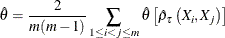The COPULA Procedure

Calibration Estimation

Instead of fitting the whole distribution as in MLE methods, you can directly use empirical estimates of distribution parameters. The unknown parameter that you want to estimate can be obtained by calibration using Kendall’s tau. There exists a one-to-one map between the parameter at interest and Kendall’s tau. Therefore, after you estimate the Kendall’s tau, you can use the map to compute the parameter value. For example, the parameter matrix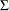in a t copula and the parameterin Archimedean copulas can be estimated in this manner. The most frequently used estimator of Kendall’s tau is the rank correlation coefficient: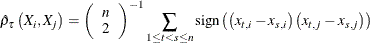The preceding formula is analogous to its population counterpart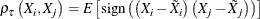where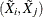has the same distribution but is independent of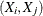.

For Archimedean multivariate copulas there is only one parameter to estimate,(or its function), although for m variables there are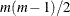unique pairwise correlation coefficients. Denote the map from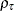toby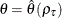. To aggregate the map, take simple arithmetic average: